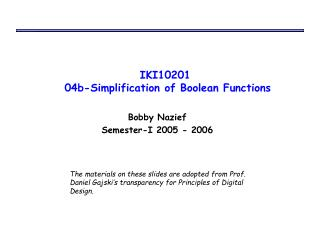DownloadDownload PresentationIKI10201 04b-Simplification of Boolean Functions

# IKI10201 04b-Simplification of Boolean Functions

Download Presentation## IKI10201 04b-Simplification of Boolean Functions

- - - - - - - - - - - - - - - - - - - - - - - - - - - E N D - - - - - - - - - - - - - - - - - - - - - - - - - - -
##### Presentation Transcript

1. IKI10201 04b-Simplification of Boolean Functions Bobby Nazief Semester-I 2005 - 2006 The materials on these slides are adopted from Prof. Daniel Gajski’s transparency for Principles of Digital Design.

2. Tabulation Method • Map method is a trial-and-error procedure • Tabulation method performs thorough search • It starts with SOM and consists of 2 steps: • PIs generation • group minterms by number of 1s • compare minterms & find pairs that differ in 1 variable • generate subcubes • repeat the above 3 steps to generate subcubes until no more subcubes can be generated • Minimal cover generation • find EPIs through a selection table • find minimal cover through the POS of PIs

3. Example: simplify w’y’z’ + wz + xyz + w’y • K-map representation: • PIs generation: • 0-subcubes yzwx

4. Example: simplify w’y’z’ + wz + xyz + w’y (cont.) • 1-subcubes • 2-subcubes

5. Example: simplify w’y’z’ + wz + xyz + w’y (cont.) • Minimal cover generation: • EPIs selection • PI list:w’z’, w’y, yz, wz • EPI list:w’z’, wz • POS:(P2 + P3)(P2 + P3) = P2 + P3 • Minimal cover expressions: • F1 = w’z’ + wz + w’y • F2 = w’z’ + wz + yz

6. Another example • K-map representation: • PIs generation: • 0-subcubes, 1-subcubes yzwx

7. Another example (cont.) • Minimal cover generation: • EPIs selection • PI list:w’yz’, x’y’z, w’xy, wx’z, xyz, wyz • EPI list:w’yz’, x’y’z • POS:(P3 + P5)(P4 + P6)(P5 + P6) =(P3 + P5)(P4P5 + P5P6 + P4P6 + P6) = P3P4P5 + P4P5 + P3P6 + P5P6 • Minimal cover expressions: • F1 = w’yz’ + x’y’z + wx’z + xyz • F2 = w’yz’ + x’y’z + w’xy + wyz • F3 = w’yz’ + x’y’z + xyz + wyz

8. Technology Mapping for Gate Arrays • Gate arrays contain only one type of m-input gate (such as 3-input NAND, 3-input NOR) • Technology mapping is a transformation of Boolean expressions into a logic schematic containing only this type (NAND or NOR) of gate • SOP/POS  NAND/NOR gate implementation

9. Conversion & Optimization • Conversion: • Optimization: • Conversion procedure: replace AND & OR gates with NAND (NOR) gates by using Rules 1 & 2 (3 & 4), and eliminate double inverters whenever possible

10. Translation standard forms to NAND/NOR schematics

11. Conversion to NAND (NOR) gates

12. Technology Mapping for Custom Libraries • Libraries contain gates with different functions and different delays • Technology mapping means covering schematic with library gates • Minimize delay on critical paths • Minimize cost on non-critical paths

13. Example design with custom libraries • F = w’z’ + z(w + y) • AND-OR implementation (delay = 7.2ns, cost = 28) • NAND implementation (delay = 5.2ns, cost = 22)

14. Example design with custom libraries (cont.) • Alternatif A (delay = 5.4ns, cost = 20) • Alternatif B (delay = 3.8ns, cost = 20) • Alternatif B-optimized (delay = 3.8ns, cost = 18)

15. Timing Diagram Design with static 1-hazard

16. Timing Diagram Hazard-free design